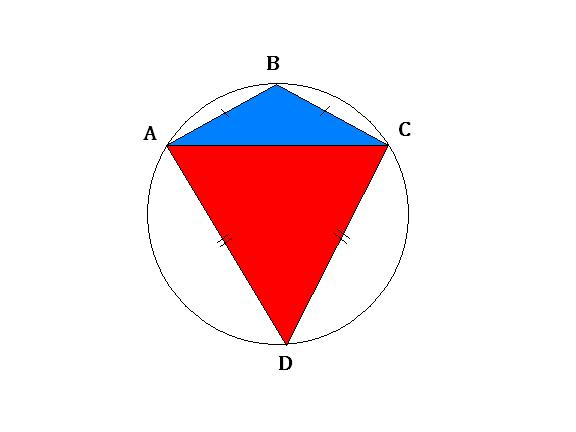# The Right Kite

Geometry Level 2Diagram above shows a kite $ABCD$ circumscribed in a circle.

If the area ratio of the red to the blue region is $3 : 1$, what is the measure of $\angle ABC$ in degrees?

Hint: If you know the right kite, things will get much easier.

×